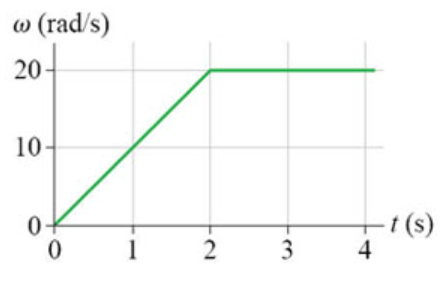# Problem: The figure shows the angular-velocity-versus-time graph for a particle moving in a circle.How many revolutions does the object make during the first 3.5 s (using appropriate units)

###### FREE Expert Solution

The number of revolutions is found by:

No. of revolutions = Δθ/2π

Δθ is the area under the angular-velocity-versus-time graph

86% (459 ratings)###### Problem Details

The figure shows the angular-velocity-versus-time graph for a particle moving in a circle.
How many revolutions does the object make during the first 3.5 s (using appropriate units)